# Designing Finite Automata from Regular Expression (Set 2)

Prerequisite: Finite automata, Regular expressions, grammar and language. Designing finite automata from Regular expression
In the below article, we shall see some Designing of Finite Automata form the given Regular Expression-

Regular Expression 1: Φ (Phi).
The language of the given RE is L1 = {} i.e, empty string.
Its finite automata will be like below-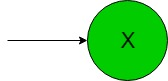In the above transition diagram, as we can see that state ‘X’ is not accepting any alphabet.

Regular Expression 2: ‘a’ (Alphabet ‘a’).
The language of the given RE is L2 = {a} i.e, a language containing only ‘a’ as the string.
Its finite automata will be like below-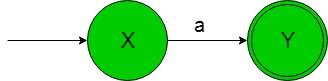In the above transition diagram, as we can see that state ‘X’ on getting ‘a’ as the alphabet it transits to a state ‘Y’. Thus it accepts only ‘a’ as the alphabet.

Regular Expression 3: ‘a+b’ (Union process of two alphabets ‘a’ and ‘b’).
The language of the given RE is L3 = {a, b} i.e, a language containing ‘a’ and ‘b’ as the string.
Its finite automata will be like below-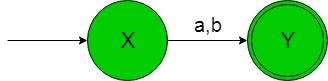In the above transition diagram, as we can see that state ‘X’ on getting either ‘a’ or ‘b’ as the alphabet it transits to a state ‘Y’. Thus it accept the desired language’s strings.

Regular Expression 4: ‘a.b’ (Concatenation of two alphabets ‘a’ and ‘b’).
The language of the given RE is L4 = {ab} i.e, a language containing ‘ab’ as the string only.
Its finite automata will be like below-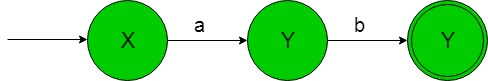In the above transition diagram, as we can see that state ‘X’ on getting ‘a’ as the alphabet it transits to state ‘Y’ and the state ‘Y’ on getting ‘b’ as the input string it transits to the final state ‘Z’. Thus it accepts the desired language’s string.

Regular Expression 5: ‘a*‘ (Kleene closure of alphabet ‘a’).
The language of the given RE is L5 = {Ε, a, aa, aaa, aaaa, ………….} i.e, a language containing Ε and any number of ‘a’.
Its finite automata will be like below-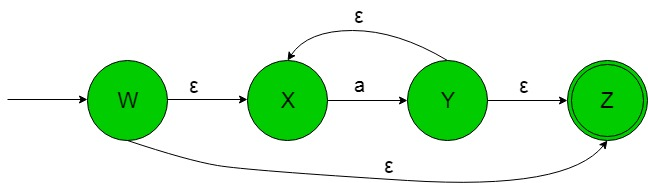In the above transition diagram, the state ‘W’ on getting Ε as the input, it transits to a final state ‘Z’ or to a state ‘X’ if the input is ‘a’. The state ‘X’ on getting ‘a’ as the input alphabet it transits to a state ‘Y’ which is connected to the final state ‘Z’ through Ε input and so on for the remaining states.
Thus above FA accepts all the language containing strings as Ε and any number of ‘a’.My Personal Notes arrow_drop_upCheck out this Author's contributed articles.

If you like GeeksforGeeks and would like to contribute, you can also write an article using contribute.geeksforgeeks.org or mail your article to contribute@geeksforgeeks.org. See your article appearing on the GeeksforGeeks main page and help other Geeks.

Please Improve this article if you find anything incorrect by clicking on the "Improve Article" button below.

Improved By : Akanksha_Rai

Article Tags :

Be the First to upvote.

Please write to us at contribute@geeksforgeeks.org to report any issue with the above content.